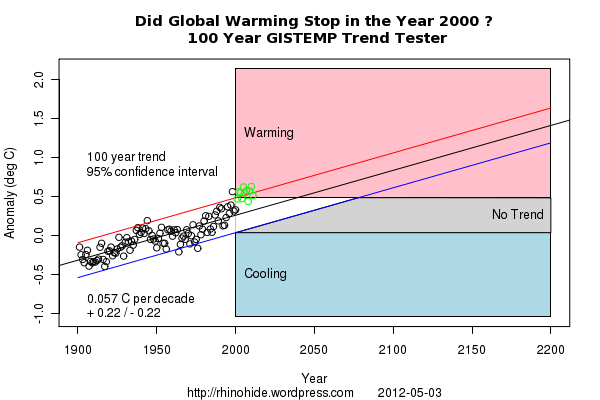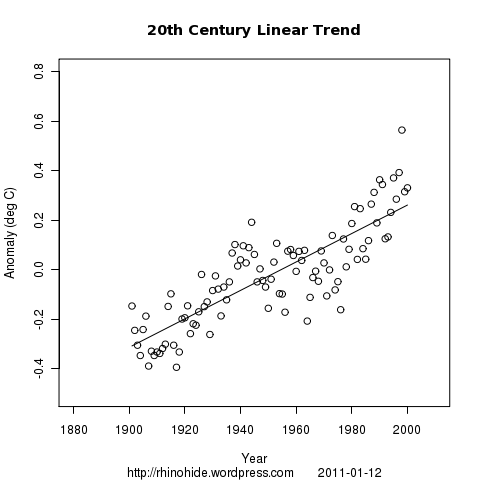Home > GIStemp, LSCF > Lines, Sines, and Curve Fitting 1 … oh my!

Lines, Sines, and Curve Fitting 1 … oh my!

2011 January 7

Looking at the 20th century data, it seems that there might be a sinusoidal signal in there some where. For instance …I’m gonna try to fit a sine wave onto a linear trend.

First, a simple linear trend on the data from 1900 to 2000Next we extract the residuals, the difference between the actual data points and the trend line, and try to fit a sinusoidal curve. I don’t know if there is a simple function for fitting sine curves. I just parameterized a sine equation, did a few loops around the parameter space, and fitted to the best correlation. The code looks like this:

for (A in 0:20) {
for (b in 20:30) {
for (T in 50:60) {
sina <- (A/100) * sin(((x-b)/T)*(2*pi))
res2 <- res - sina
ss <- sum(res2^2)
s <- rbind(s,cbind(A,b,T,ss))
}}}
#s[s[,4]==min(s[,4],na.rm=T),]
A <- s[s[,4]==min(s[,4],na.rm=T),1]
b <- s[s[,4]==min(s[,4],na.rm=T),2]
T <- s[s[,4]==min(s[,4],na.rm=T),3]

And the sine fit to the residuals looks like this:Now, just to add them together like this:How good is the fit?
The correlation of the linear trend is 0.83.
The correlation of the sine to the residuals is 0.86.
And the correlation of the line and sine to the original data is 0.89.

What are the parameters?
The slope (m) of the linear trend is 0.57 degC per century.
The amplitude (A) of the sine is 0.08C.
The phase shift (b) is 24 years.
And the period (T) is 56 years.

The script is here.